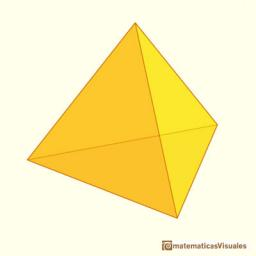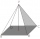# Triangular pyramid

Calculate the volume and surface area of a regular triangular pyramid with height equal to the base edge 10 cm length.

V =  144.3376 cm3
S =  193.3638 cm2

### Step-by-step explanation:Did you find an error or inaccuracy? Feel free to write us. Thank you!

Showing 1 comment:Reynie
Easy problem solving????????????????????????Tips to related online calculators
Do you want to convert length units?
Tip: Our volume units converter will help you with the conversion of volume units.
Pythagorean theorem is the base for the right triangle calculator.

#### You need to know the following knowledge to solve this word math problem:

We encourage you to watch this tutorial video on this math problem:

## Related math problems and questions:

• Triangular pyramidDetermine the volume and surface area of a regular triangular pyramid having a base edge a=20 cm and a lateral edge b = 35 cm
• Hexagonal pyramidCalculate the volume and the surface of a regular hexagonal pyramid with a base edge length of 3 cm and a height of 5 cm.
• Pyramid heightFind the volume of a regular triangular pyramid with edge length a = 12cm and pyramid height h = 20cm.We have a regular quadrilateral pyramid with a base edge a = 10 cm and a height v = 7 cm. Calculate 1/base content 2/casing content 3/pyramid surface 4/volume of the pyramid
• Triangular pyramidCalculate the volume of a regular triangular pyramid with edge length a = 12cm and pyramid height v = 20cm.Find the height and surface of a regular quadrilateral pyramid with a base edge a = 8cm and a wall height w = 10cm. Sketch a picture.
• Pyramid 4sidesCalculate the volume and the surface of a regular quadrangular pyramid when the edge of the base is 4 cm long and the height of the pyramid is 7 cm.
• Hexagonal pyramidFind the area of a shell of the regular hexagonal pyramid, if you know that its base edge is 5 cm long and the height of this pyramid is 10 cm.Find the volume and surface of a regular quadrilateral pyramid if the bottom edge is 45 cm long and the pyramid height is 7 cm.
• Triangular pyramidIt is given perpendicular regular triangular pyramid: base side a = 5 cm, height v = 8 cm, volume V = 28.8 cm3. What is it content (surface area)?
• Triangular prismThe base of the perpendicular triangular prism is a right triangle with a leg length of 5 cm. The content area of the largest sidewall of its surface is 130 cm², and the height of the body is 10 cm. Calculate its volume.
• Regular hexagonal pyramidCalculate the height of a regular hexagonal pyramid with a base edge of 5 cm and a wall height of w = 20cm. Sketch a picture.
• Tetrahedral pyramidIt is given a regular tetrahedral pyramid with a base edge of 6 cm and the height of the pyramid 10 cm. Calculate the length of its side edges.
• Tetrahedral pyramidCalculate the volume and surface area of a regular tetrahedral pyramid, its height is \$b cm and the length of the edges of the base is 6 cm.The regular quadrangular pyramid has a base length of 6 cm and a side edge length of 9 centimeters. Calculate its volume and surface area.Calculate the surface of a quadrilateral pyramid, which has a rectangular base with dimensions a = 8 cm, b = 6 cm and height H = 10 cm.Calculate the pyramid's volume and surface area with the edge and height a = 26 cm. h = 3 dm.